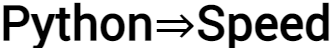# float64 to float32: Saving memory without losing precisionLibraries like NumPy and Pandas let you switch data types, which allows you to reduce memory usage.
Switching from `numpy.float64` (“double-precision” or 64-bit floats) to `numpy.float32` (“single-precision” or 32-bit floats) cuts memory usage in half.
But it does so at a cost: `float32` can only store a much smaller range of numbers, with less precision.

So if you want to save memory, how do you use `float32` without distorting your results?
Let’s find out!

In particular, we will:

• Explore some of the limits of the numbers `float32` lets you express.
• Discuss a couple of different ways to solve the problem using basic arithmetic.
• Suggest a different solution to reducing memory, which gives you an even bigger range than